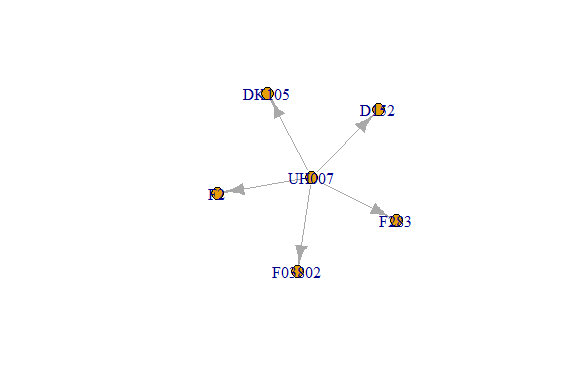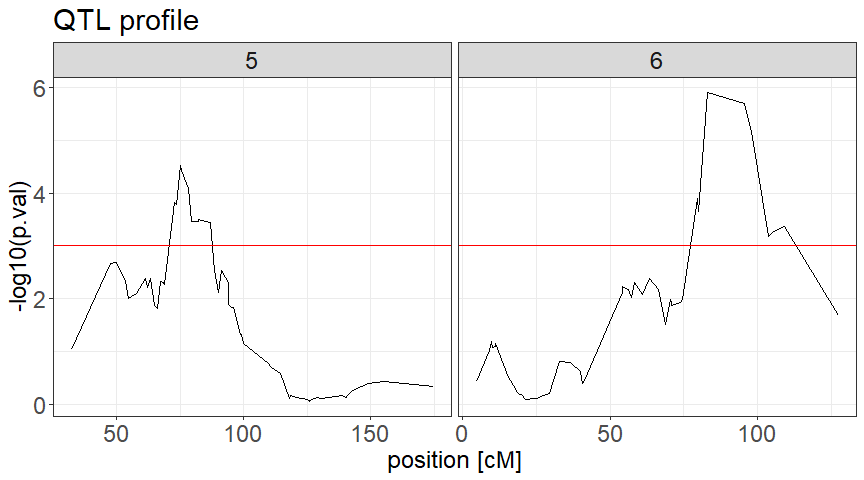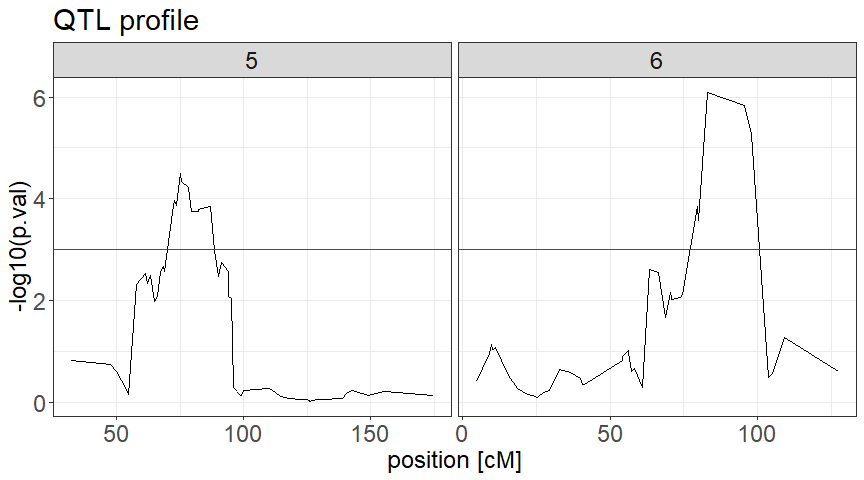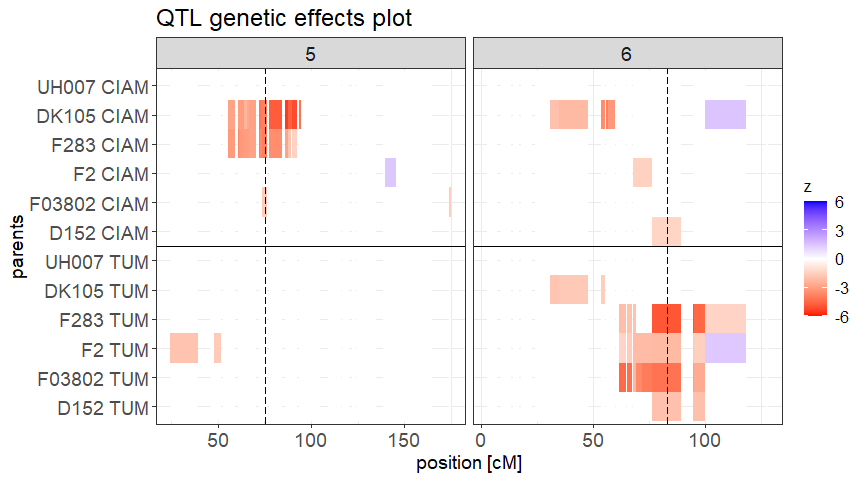# QTL detection in multiparental populations characterized in multiple environments

## Multiparental populations

Multiparental populations (MPPs) like the nested association mapping (NAM; McMullen et al. (2009)) or multi-parent advanced generation inter-cross (MAGIC; Cavanagh et al. (2008)) populations have emerged as central genetic resources for research and breeding purposes . The diallels , the factorial designs or the collections of crosses developed in breeding programs can also be considered as MPPs. We can classify MPPs given the use of intrecrossing. In a first type of MPPs, there is no intercrossing. Each genotype inherits its alleles from two parents. Those MPPs can be seen as a collection of crosses (e.g. NAM or factorial design). A second type of MPPs like MAGIC involve genotypes that are a mosaic of more than two parents or founders. In this package, we only consider the first type of MPPs without intercrossing or collections of crosses with shared parents.

## MPP-ME QTL detection

Even though several statistical methodologies and software are available to perform QTL detection in MPPs, there is potentially few or no open-source solution for the detection of QTL in MPPs characterized in multiple environments (MPP-ME). In this extension of mppR, we propose a method to detect QTLs in MPP-ME data.

## Model description

We start from the QTL detection model 3 described in :

$\underline{y}_{icj} = \mu + e_{j} + c_{cj} + x_{ip} * \beta_{pj} + \underline{ge}_{icj} + \underline{\epsilon}_{icj} \quad $

where $$\underline{y}_{icj}$$ is the genotype mean (BLUE) of genotype $$i$$ from cross $$c$$ in environment $$j$$. $$e_{j}$$ is a fixed environment term, $$c_{cj}$$ a fixed cross within environment interaction, $$x_{ip}$$ is the number of allele copies coming from parent $$p$$, and $$\beta_{pj}$$ represent the QTL effect of parental allele $$p$$ in environment $$j$$. Different definitions of the QTL allelic effect are possible . In this version of the model we assumed that each parent carries a different allele and that each of those alleles can have an environmental specific effect. The model is therefore saturated with the estimation of $$N_{env} * N_{par}$$ effects with one parent (the most frequently used) set as the reference in all environments (e.g. the central or recurrent parent in NAM).

The term $$ge_{icj}$$ is the residual genetic variation and $$\epsilon_{icj}$$ the plot error term. In model 1 because genotype values are averaged over replication $$\epsilon_{icj}$$ and $$ge_{icj}$$ are confounded. The variance of the $$(ge_{icj} + \epsilon_{icj})$$ term can be modeled with different variance covariance (VCOV) structures. A first possibility is to assume a constant genotypic variance across environments $$\sigma_{g}^{2}$$ (compound symmetry - CS) and a unique variance for the plot error term $$\sigma_{\epsilon}^{2}$$:

$V\begin{bmatrix} y_{i11} \\ y_{i'21} \\ y_{i12} \\ y_{i'22} \\ y_{i13} \\ y_{i'23} \end{bmatrix} = \begin{bmatrix} \sigma_{g}^{2} + \sigma_{\epsilon}^{2} & 0 & \sigma_{g}^{2} & 0 & \sigma_{g}^{2} & 0 \\ 0 & \sigma_{g}^{2} + \sigma_{\epsilon}^{2} & 0 & \sigma_{g}^{2} & 0 & \sigma_{g}^{2} \\ \sigma_{g}^{2} & 0 & \sigma_{g}^{2} + \sigma_{\epsilon}^{2} & 0 & \sigma_{g}^{2} & 0 \\ 0 & \sigma_{g}^{2} & 0 & \sigma_{g}^{2} + \sigma_{\epsilon}^{2} & 0 & \sigma_{g}^{2} \\ \sigma_{g}^{2} & 0 & \sigma_{g}^{2} & 0 & \sigma_{g}^{2} + \sigma_{\epsilon}^{2} & 0 \\ 0 & \sigma_{g}^{2} & 0 & \sigma_{g}^{2} & 0 & \sigma_{g}^{2} + \sigma_{\epsilon}^{2} \\ \end{bmatrix}$

The CS model requires the estimation of a single term ($$\sigma_{g}^{2}$$) to describe genetic the covariance between all pairs of environments. This model assumes that the background polygenic effect (effect of all genome positions except the tested QTL and cofactors) is the same in all environments .

A more realistic option called unstructured (UN) model allows the genetic covariance to be different in every pairs of environments. From a genetic point of view, it means that a set of genes have a different effect in each environments . In the unstructured model, each pair of environment get a specific genetic covariance term $$cov(y..j, y..j') = \sigma_{g_{jj'}}^{2}$$

$V\begin{bmatrix} y_{i11} \\ y_{i'21} \\ y_{i12} \\ y_{i'22} \\ y_{i13} \\ y_{i'23} \end{bmatrix} = \begin{bmatrix} \sigma_{g_{1}}^{2} + \sigma_{\epsilon}^{2} & 0 & \sigma_{g_{12}} & 0 & \sigma_{g_{13}} & 0 \\ 0 & \sigma_{g_{1}}^{2} + \sigma_{\epsilon}^{2} & 0 & \sigma_{g_{12}} & 0 & \sigma_{g_{13}} \\ \sigma_{g_{12}} & 0 & \sigma_{g_{2}}^{2} + \sigma_{\epsilon}^{2} & 0 & \sigma_{g_{23}} & 0 \\ 0 & \sigma_{g_{12}} & 0 & \sigma_{g_{2}}^{2} + \sigma_{\epsilon}^{2} & 0 & \sigma_{g_{23}} \\ \sigma_{g_{13}} & 0 & \sigma_{g_{23}} & 0 & \sigma_{g_{3}}^{2} + \sigma_{\epsilon}^{2} & 0 \\ 0 & \sigma_{g_{13}} & 0 & \sigma_{g_{23}} & 0 & \sigma_{g_{3}}^{2} + \sigma_{\epsilon}^{2} \\ \end{bmatrix}$

We considered the UN model as the default option.

## Approximate Wald statistic computation

The calculation of an ‘exact’ mixed model at each marker (QTL) position of the genome requires a lot of computer power, which can quickly make detection unfeasible, especially with large populations. Therefore, to reduce the computational power needed for a genome scan, we implemented an approximate mixed model computation similar to the generalized least square strategy implemented in Kruijer et al. (2015) or Garin et al. (2017). The procedure consists of estimating a general VCOV ($$\hat{V}$$) using model 1 without the tested QTL position for the simple interval mapping (SIM) scan. For the composite interval mapping (CIM) model, $$\hat{V}$$ is estimated including the cofactors as fixed effect without the QTL position. For the CIM scan, there will be as many $$\hat{V}$$ as the number of cofactors combinations given cofactor exclusion around the tested position.

There are two option for the estimation of $$\hat{V}$$. In the first option we estimate a unique $$\hat{V}$$ taking all cofactors into consideration. In the second option, different $$\hat{V}$$ should be formed by removing the cofactor information that is too close of a tested QTL position.

The statistical significance of the tested QTL position and the allelic effects is obtained by substituting $$\hat{V}$$ to get the following Wald statistic $$W_Q = \beta^{T} [V(\beta)]^{-1} \beta \quad $$, where $$\beta = (X^{T} \hat{V}^{-1} X)^{-1} X^T \hat{V}^{-1} y \quad $$, $$V(\beta) = (X^{T} \hat{V}^{-1} X)^{-1}$$, $$X$$ represents the fixed effect matrix including the cross effect, the cofactors, and the QTL position, and $$y$$ is the vector of genotypic values. $$W_Q \sim \chi_{d}^{2}$$ with degree of freedom ($$d$$) equal to the number of tested QTL allelic effects ($$(N_{par}-1) * N_{env}$$) for the main QTL term or one for each specific within environment allele).

## QTL effects estimation

In model 1, QTL terms are considered as fixed. Therefore, the user needs to provide best linear unbiased estimates (BLUEs) as trait value. Beyond estimating a general QTL significance, model 1 allows the estimation of QTL allelic effect for each parents in each environment. One parent is set as reference. By default in populations like the nested association mapping (NAM) populations the central parent is considered as the reference. For other types of MPPs the reference parent can be specified using a dedicated function argument (ref_par). The parental QTL allelic effect must be interpreted as the extra effect of one allele copy with respect to the reference parent in the considered environment.

## QTL by environmental covariate (EC) effect estimation

For QTLs showing a significant QTL by environment (QTLxE) effects, it is possible to investigate which specific dimension of the environment (environmental covariate - EC) explains the QTLxE effect. Therefore, we propose a strategy to estimate the sensitivity of the parental QTL allele to a specific EC (rain, temperature, photoperiod, etc.). For that purpose, we extended the QTL term of model 1 like that $$x_{ip} * \beta_{pj} = x_{ip} * (\beta_{p} + EC_{e}*S_{p} + l_{pe})$$ where $$\beta_{p}$$ is the main parental allelic effect across environments, $$EC_{e}$$ is the EC value in each environment, $$S_{p}$$ is the parental allele sensitivity to $$EC_{e}$$ change and $$l_{pe}$$ the residual effect. The $$S_{p}$$ determine the rate of change of the parental QTL allelic additive effect given an extra unit of EC. The significance of $$S_{p}$$ allows to assess the degree of sensitivity of the QTL to the EC. To avoid model saturation, we implemented a procedure that first estimates the QTL parental alleles effect across (main effect term) and between environment (QTLxE term). Then, the function compare the significance of those two terms. The QTL by EC (QTLxEC) is only estimated for the parental allelic effect for which the QTLxE term is more significant than the main effect term.

## QTL detection procedure overview

The function mppGE_proc() perform the whole procedure detailed here:

1. SIM scan
2. Selection of cofactors
3. CIM scan
4. Selection of QTLs
5. Estimation of the QTL allele effects
6. Estimation of the QTL R2 contribution
7. Plot of the QTL profile
8. Visualisation of the allelic significance along the genome

## Procedure detail and examples

### Data

The format of the data is the same as the default format used by mppR (mppData objects). The trait measured in the different environments are simply added as separate columns of the pheno argument when you create the mppData object with create.mppData().

The data used in this vignette (mppData_GE) come from the EU-NAM Flint population . The genotype data come from five crosses between the donor parents: D152, F03802, F2, F283, DK105 with the recurrent parent UH007. We selected a subset of 100 markers spread over chromosomes five and six. The genetic map was calculated by Giraud et al. (2014). The phenotypic data represent the within environment adjusted means for dry matter yield (DMY) calculated at La Coruna (CIAM), Roggenstein (TUM), Einbeck (KWS), and Ploudaniel (INRA_P) Lehermeier et al. (2014).

library(mppR)
data(mppData_GE)
design_connectivity(par_per_cross = mppData_GE$par.per.cross)#>$1
#>  "UH007"  "D152"   "F03802" "F2"     "F283"   "DK105"

### Simple interval mapping (SIM)

To perform the SIM scan, you simply need to pass the mppData object to the function. and specify the environments you want to use in the trait argument. If needed, a reference parent can be specified using the ref_par argument. For example, here analyse the trait DMY characterized at CIAM and TUM. After calculation, the SIM profile can be ploted with plot()

SIM <- mppGE_SIM(mppData = mppData_GE, trait = c('DMY_CIAM', 'DMY_TUM'), ref_par = 'UH007')
plot(x = SIM)### Cofactors selection

The cofactors as well as the QTLs are selected using the same function (QTL_select()). It performs an iterative search per chromosome starting from the most significant position above the threshold value. Then, marker positions falling on the left and right of the selected position by a distance specified in window are excluded. The search continues with the remaining positions by repeating the selection and exclusion steps. The search stops when no position remains above the threshold.

The default value of window (50) is deliberately large to limit the number of included cofactors and not overfit the model. In the mppGE_proc() function, the cofactor threshold and window parameters can be modified through the thre.cof and win.cof arguments.

cofactors <- QTL_select(Qprof = SIM, threshold = 4, window = 50)

### Composite interval mapping (CIM)

To perform the CIM scan, you simply need to pass the selected cofators to the mppGE_CIM() function. The window parameter specifies the distance on the left and right where cofactors are excluded in the neighborhood of a tested position. The VCOV_data argument allow to specify if the user want to estimate a unique general VCOV with all cofactors included (“unique”) or several VCOV removing cofactor in the neighbouring of a QTL position (“minus_cof”). The unique option is faster.

CIM <- mppGE_CIM(mppData = mppData_GE, trait = c('DMY_CIAM', 'DMY_TUM'),
VCOV = "UN", VCOV_data = "unique", cofactors = cofactors,
window = 20)

### QTLs selection

The selection of QTLs is done using the same function as the one used for cofactors. For the selection of QTLs it is possible to restrict the size of the exclusion window around the selected positions. In the mppGE_proc() function, the QTL threshold and window parameters can be modified through the thre.QTL and win.QTL arguments.

QTL <- QTL_select(Qprof = SIM, threshold = 4, window = 20)

### QTLs effect and significance estimation

The function QTL_effect_GE() allows the estimation of the QTL allele effects and their significance. It calculates an exact version of mixed model 1 with single or multiple QTLs. The QTL allele effects are estimated using  and their significance with the Wald test .

The results of the QTL allelic effect estimation are returned in a list where each component represents the effects of one QTL. The individual within environment QTL effects are represented in row while the effects ($$\hat{\beta}$$), their standard error, as well as their significance are represented in columns. For example, looking at the first QTL, we can see that in the first environment the the allele of parent DK105 decreases the DMY by -9.861 deciton/ha compared to the central parent UH007. In the second environment, the effect of DK105 is only -1.559. The effect is highly significant in the first environment but not in the second.

Qeff <- QTL_effect_GE(mppData = mppData_GE, trait = c('DMY_CIAM', 'DMY_TUM'),
QTL = QTL)
Qeff$QTL_1 #> Effect Std.dev Wald df p.val sign #> QTL_1_UH007_E1 NA NA NA NA NA <NA> #> QTL_1_D152_E1 0.979 3.107 0.12 1 0.7238207754 #> QTL_1_F03802_E1 -4.632 2.265 4.98 1 0.0256716793 * #> QTL_1_F2_E1 -3.170 3.523 0.97 1 0.3250043320 #> QTL_1_F283_E1 -7.691 2.189 14.75 1 0.0001228353 *** #> QTL_1_DK105_E1 -9.861 2.615 17.11 1 0.0000352697 *** #> QTL_1_UH007_E2 NA NA NA NA NA <NA> #> QTL_1_D152_E2 2.351 3.010 0.77 1 0.3792850449 #> QTL_1_F03802_E2 1.921 2.173 0.93 1 0.3344656579 #> QTL_1_F2_E2 -3.200 3.386 1.07 1 0.3010936767 #> QTL_1_F283_E2 -0.561 2.110 0.08 1 0.7715930671 #> QTL_1_DK105_E2 -1.559 2.513 0.46 1 0.4961859621 A specific parent can be selected as reference using the ref_par argument. Qeff <- QTL_effect_GE(mppData = mppData_GE, trait = c('DMY_CIAM', 'DMY_TUM'), QTL = QTL, ref_par = 'F2') Qeff$QTL_1
#>                 Effect Std.dev Wald df       p.val sign
#> QTL_1_UH007_E1   3.170   3.523 8.88  1 0.002882220   **
#> QTL_1_D152_E1    4.149   4.697 2.24  1 0.134327711
#> QTL_1_F03802_E1 -1.462   4.188 0.50  1 0.481184513
#> QTL_1_F2_E1         NA      NA   NA NA          NA <NA>
#> QTL_1_F283_E1   -4.522   4.148 5.10  1 0.023958287    *
#> QTL_1_DK105_E1  -6.691   4.387 7.88  1 0.005002411   **
#> QTL_1_UH007_E2   3.200   3.386 9.78  1 0.001761073   **
#> QTL_1_D152_E2    5.552   4.530 4.31  1 0.037904061    *
#> QTL_1_F03802_E2  5.122   4.023 6.62  1 0.010087175    *
#> QTL_1_F2_E2         NA      NA   NA NA          NA <NA>
#> QTL_1_F283_E2    2.640   3.989 1.87  1 0.171700270
#> QTL_1_DK105_E2   1.641   4.217 0.51  1 0.473716237

### QTL R2 calculation

The function QTL_R2_GE() allows the estimation of an $$R^{2}$$ contribution of all the QTLs (global) and of each QTL positions (partial). For simplicity, the $$R^{2}$$ is estimated using a linear model version of  and not a mixed model, which means that the $$ge_{icj}$$ term is absent from the model and that only a unique variance error term $$\sigma_{\epsilon}^{2}$$ is estimated. The $$R^{2}$$ values represent therefore general tendencies and should be taken with caution.

The global adjusted $$R^{2}$$ is defined as $$R^{2} = 100*(1-(\frac{RSS_{full}/d_{full}}{RSS_{red}/d_{red}}))$$, where $$RSS_{full}$$ and $$RSS_{red}$$ are the residual sum of squares of the model with and without the QTL positions. $$d_{full}$$ and $$d_{red}$$ represent the degree of freedom of the corresponding models. The partial (adjusted) $$R^{2}$$ of QTL $$i$$ is the difference between the global $$R^{2}$$ obtained with all QTLs and the $$R^{2}$$ obtained with a model minus QTL $$i$$.

QR2 <- QTL_R2_GE(mppData = mppData_GE, trait = c('DMY_CIAM', 'DMY_TUM'), QTL = QTL)

QR2$glb.adj.R2 #>  6.328735 QR2$part.adj.R2.diff
#>       Q1       Q2
#> 2.563632 3.582575

### QTL profile plot

The profile plot of the QTL significance can be obtained using the plot.QTLprof() function that plots the $$-log_{10}(p-val)$$ of the position along the genome.

plot(x = CIM)### Whole-genome genetic effect significance plot

It is also possible to visualize the significance of the QTL parental allele along the by passing the QTL profile information (CIM) to plot_Qeff_prof() function. The QTL argument can be used to specify potential QTL positions.

The color intensity $$z = -log10(p-val)$$ is proportional to the statistical significance of the parental allelic effects obtained from the Wald test . $$z$$ has an upper limit of six to not let extreme signficant value influence too much the color scale. Compared to the reference parent (top of the graph) the red (blue) colour represents a negative (positive) effect. The different panels from the top to the bottom represent the different environments.

plot_allele_eff_GE(mppData = mppData_GE, nEnv = 2,
EnvNames = c('CIAM', 'TUM'), Qprof = CIM,
QTL = QTL, text.size = 14)### mppGE_proc: wrapper function

It is possible to calculate the whole procedure described using the unique mppGE_proc() function. This function uses the same arguments as the one defined in the sub-functions (e.g. mppData, trait, thre.cof, win.cof, window, ref_par etc.). In this function it is possible to specify a working directory in output.loc. A folder with the pop.name and trait.name will be created to store intermediate data output like the SIM and CIM profiles, some results (list of QTLs, plots), as well as a summary of the results (QTL_REPORT.txt). Here we can still introduce the argument n.cores which allow to run the function in parallel on multiple cores.

MPP_GE_QTL <- mppGE_proc(pop.name = 'EUNAM', trait.name = 'DMY',
mppData = mppData_GE, trait = c('DMY_CIAM', 'DMY_TUM'),
n.cores = 1, verbose = FALSE, output.loc = tempdir())

### QTLxEC effect estimation

Once significant QTL effects have been determined, it is possible to investigate if the parental QTL allelic effect interact with specific dimension of the environment (environmental covariate - EC) like rain, temperature, etc. For that purpose you can use the QTL_effect_QxEC(). This function first calculates the signficance of the parental QTL alleles accross environment (main effect term) and the interaction with the environment (QTLxE term) using the sub-function QTL_effect_main_QxE(). Then, it only calculate the QTL effect sensitivity to the EC for the parental allelic effect that show a larger significance of the QTLxE term compared to the main term. To calculate such sensitivity, the user need to provide a list of QTL as well as EC value. The EC value take the form of a matrix with environment in row and ECs in columns. It is possible to estimate the effect of several ECs but user should be careful to have enough degrees of freedom (df) to estimate those sensitivity curve. For example, with four environments, if estimate the effect of a unique EC, you only have two remaining df (4 env - 1 df main effect - 1 df sensitivity slope).

EC <- matrix(c(180, 310, 240, 280), 4, 1)
rownames(EC) <- c('CIAM', 'TUM', 'INRA', 'KWS')
colnames(EC) <- 'cum_rain'

Qeff <- QTL_effect_QxEC(mppData = mppData_GE,
trait = c('DMY_CIAM', 'DMY_TUM', 'DMY_INRA_P', 'DMY_KWS'),
env_id = c('CIAM', 'TUM', 'INRA', 'KWS'),
QTL = QTL, EC = EC)

Qeff$Qeff_EC$QTL1

The results take the form of a list. The first element gives the result of the comparison between main effect and QTLxE term significance expressed in $$-log10(p-value)$$. The second term return the estimated main allelic effect as well as the QTL allelic sensitivity and its significance in the $$-log10(p-value)$$ scale. The parental QTL allelic sensitivity can be plotted using the function plot_QTLxEC(). The plot represent the sensitivity curve of the significant parental allelic effect with respect to the reference parent.

pl <- plot_QTLxEC(Qeff, Q_id = 2, RP = "UH007", EC_id = 'cum rain', trait_id = 'DMY')
pl

### Computation time

We tested the proposed procedure on several sorghum BCNAM populations as well as maize NAM population and breeding MPPs. The table below show the computation time required for different configurations. Computations were realized on an AMD Ryzen 7-cores 5800 U processor with 16 GB RAM.

Computation time examples
Populations Ngeno Nmarker Nenv Ncore SIM [m] CIM [h] QTL_effects [m] Total [h]
BCNAM Grinkan 1598 51545 4 4 20.0 11.00 15.0 11.60
BCNAM Kenin-Keni 575 51545 4 4 1.5 0.17 1.5 0.25
BCNAM Lata 896 51545 3 4 3.0 0.33 2.0 0.40
EUNAM Dent 841 18621 4 1 18.0 9.50 19.0 10.20
EUNAM Flint 811 5949 6 1 15.0 16.00 150.0 19.00
Breeding pop1 2071 1812 3 1 5.0 1.30 9.0 1.50
Breeding pop2 820 1760 5 1 9.0 10.20 33.0 10.80

From a general point of view, even though we tried our best to speed up the computation, it can still take several hours to perform a complete analysis. The computation time first depends on the population size (number of genotypes), the number of environments, as well as the number of markers. The use of multiple cores (n.cores argument) is a first possibility to reduce the computational time.

The computation of the CIM part including cofactors is the most demanding. The whole procedure computational time can be largely reduced if the user only perform a SIM (SIM_only = TRUE in mppGE_proc()). Generally, the large effect QTLs are the same or fall in similar region in the SIM and CIM analysis. The number of cofactor position selected will also considerably extend the computational time. For that reason we advise to restrict their number by selecting maximum one cofactor per chrosome.

Ultimately, even if the computation time can be long we should always put that in balance with the time it required to develop the population, phenotype it and get the genotypic data. Getting quality estimation of the genetic effect is a good way to values those investments.